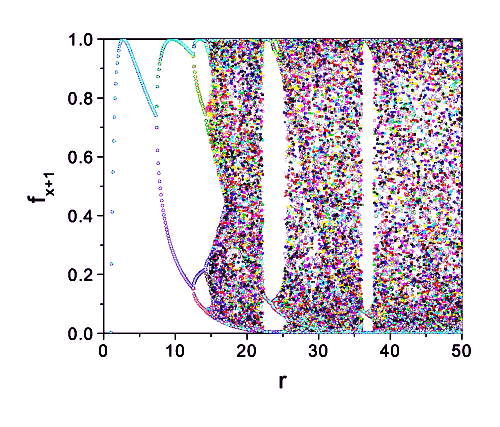Skip Navigation Links.

### Bifurcation DiagramBifurcation diagram for the map f(x+1) = r*exp(-x/k). This equation can be considered a generalization of the logistic map f(x+1) = r*x*(1-x). It is used in population studies under conditions of random migration. The first equation was used by W. E. Ricker to describe fish population dynamics. If we define r as the ratio between birth and death, and x is the normalized population estimate, we can see the model predicts stability for 1 < r < 7.25, oscillation with periodicity of two for 7.25 < r < 12.5, and chaotic behavior for r > 15, with some regions of stability.